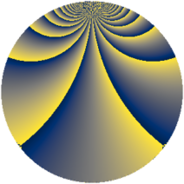# Properties

 Label 1344.4.chLevel $1344$ Weight $4$ Character orbit 1344.ch Rep. character $\chi_{1344}(139,\cdot)$ Character field $\Q(\zeta_{16})$ Dimension $3072$ Sturm bound $1024$

# Related objects

## Defining parameters

 Level: $$N$$ $$=$$ $$1344 = 2^{6} \cdot 3 \cdot 7$$ Weight: $$k$$ $$=$$ $$4$$ Character orbit: $$[\chi]$$ $$=$$ 1344.ch (of order $$16$$ and degree $$8$$) Character conductor: $$\operatorname{cond}(\chi)$$ $$=$$ $$448$$ Character field: $$\Q(\zeta_{16})$$ Sturm bound: $$1024$$

## Dimensions

The following table gives the dimensions of various subspaces of $$M_{4}(1344, [\chi])$$.

Total New Old
Modular forms 6176 3072 3104
Cusp forms 6112 3072 3040
Eisenstein series 64 0 64

## Trace form

 $$3072q + O(q^{10})$$ $$3072q + 944q^{22} + 1520q^{28} + 2000q^{44} - 11424q^{50} - 4896q^{60} + 12096q^{64} + 1632q^{67} - 1792q^{71} + 5264q^{74} - 14112q^{78} + O(q^{100})$$

## Decomposition of $$S_{4}^{\mathrm{new}}(1344, [\chi])$$ into newform subspaces

The newforms in this space have not yet been added to the LMFDB.

## Decomposition of $$S_{4}^{\mathrm{old}}(1344, [\chi])$$ into lower level spaces

$$S_{4}^{\mathrm{old}}(1344, [\chi]) \cong$$ $$S_{4}^{\mathrm{new}}(448, [\chi])$$$$^{\oplus 2}$$